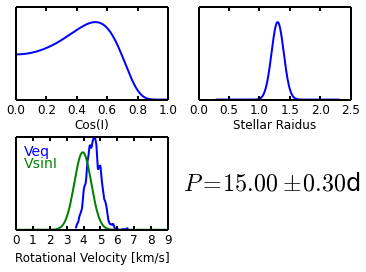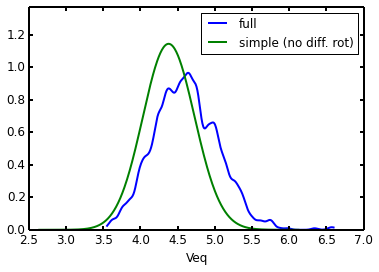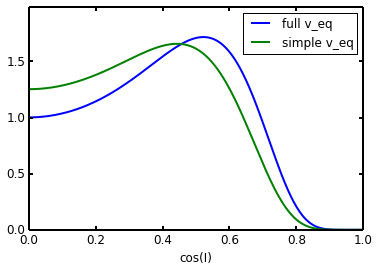In :
%pylab inline
from obliquity.distributions import Cosi_Distribution

Populating the interactive namespace from numpy and matplotlib


Here's making an example $\cos I$ posterior object. This is doing the full calculation including differential rotation, so it takes a few seconds.

In :
cosi_dist = Cosi_Distribution((1.3,0.1),(15,0.3),(3.5,0.5))
cosi_dist.summary_plot()Now, let's do it again, this time using a simple $V_{eq}$ calculation, without differential rotation.

In :
cosi_dist_simple = Cosi_Distribution((1.3,0.1),(15,0.3),(3.5,0.5),veq_simple=True) #veq_simple gives faster calculation.


Now, compare the two calculations.

In :
cosi_dist.veq_dist.plot(label='full')
cosi_dist_simple.veq_dist.plot(fig=0,label='simple (no diff. rot)',color='g')
legend()

cosi_dist.plot(label='full v_eq')
cosi_dist_simple.plot(fig=0,color='g',label='simple v_eq')
legend()

Out:
<matplotlib.legend.Legend at 0x7f8ab1ef3c10>So there's a slight difference. Correcting for differential rotation makes the estimated $V_{eq}$ a bit larger.

In :


In [ ]: